Rigid Body Dynamics Library
Constraint Class Referenceabstract

Interface to define general-purpose constraints. More...

#include <Constraint.h>Inheritance diagram for Constraint:

## Public Member Functions

virtual void bind (const Model &model)=0
Any local memory that has a dimension of N, where N is the length of QDot, is resized in this function.
More...

virtual void calcConstraintJacobian (Model &model, const double time, const Math::VectorNd &Q, const Math::VectorNd &QDot, Math::MatrixNd &GSysOutput, ConstraintCache &cache, bool updateKinematics=false)=0
In this function the matrix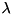of this contraint is inserted into the system constraint Jacobian(GSysOutput). The submatrixbegins at rowInSystem, has sizeOfConstraint number of rows, and fills the full number of columns in G. More...

virtual void calcGamma (Model &model, const double time, const Math::VectorNd &Q, const Math::VectorNd &QDot, const Math::MatrixNd &GSys, Math::VectorNd &gammaSysOutput, ConstraintCache &cache, bool updateKinematics=false)=0
In this function the vector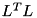of this constraint is inserted into the right-hand-side vector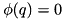of the system. The vectorbegins at rowInSystem, and has sizeOfConstraint rows. More...

virtual void calcPositionError (Model &model, const double time, const Math::VectorNd &Q, Math::VectorNd &errSysOutput, ConstraintCache &cache, bool updateKinematics=false)=0
In this function the sub vectorof this constraint is inserted into the position error vector of the system. If the constraint is velocity-level constraint or higher (noted in the boolean member variable positionConstraint) this should be set to zero. The vectorbegins at rowInSystem, and has sizeOfConstraint rows. More...

virtual void calcVelocityError (Model &model, const double time, const Math::VectorNd &Q, const Math::VectorNd &QDot, const Math::MatrixNd &GSys, Math::VectorNd &derrSysOutput, ConstraintCache &cache, bool updateKinematics=false)=0
In this function the sub vector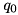of this constraint is inserted into the position error vector of the system. If the constraint is an acceleration-level constraint (noted in the boolean member variable velocityConstraint) this sub vector should be zero. The vectorbegins at rowInSystem, and has sizeOfConstraint rows. More...

virtual void calcConstraintForces (Model &model, const double time, const Math::VectorNd &Q, const Math::VectorNd &QDot, const Math::MatrixNd &GSys, const Math::VectorNd &LagrangeMultipliersSys, std::vector< unsigned int > &constraintBodiesOutput, std::vector< Math::SpatialTransform > &constraintBodyFramesOutput, std::vector< Math::SpatialVector > &constraintForcesOutput, ConstraintCache &cache, bool resolveAllInRootFrame=false, bool updateKinematics=false)=0
This function resolves the generalized forces this constraint applies to the system into the wrenches that are applied to the bodies that are involved in the constraint. More...

virtual ~Constraint ()

Constraint ()

Constraint (const char *nameOfConstraint, unsigned int typeOfConstraint, unsigned int sizeOfConstraint, unsigned int userDefinedIdNumber)

void addToConstraintSet (const unsigned int rowIndex)
This function is called by the functions in ConstraintSet that add a Constraint to the system. DO NOT TOUCH THIS. More...

unsigned int getUserDefinedId ()

void setUserDefinedId (unsigned int userDefinedId)

void getConstraintJacobian (const Math::MatrixNd &GSys, Math::MatrixNd &GConstraint)

void getGamma (const Math::VectorNd &gammaSys, Math::VectorNd &gammaConstraint)

void getPositionError (const Math::VectorNd &errSys, Math::VectorNd &errConstraint)

void getVelocityError (const Math::VectorNd &derrSys, Math::VectorNd &derrConstraint)

void getBaumgarteStabilizationParameters (Math::Vector2d &bgParamsUpd)

void getBaumgarteStabilizationForces (const Math::VectorNd &errPos, const Math::VectorNd &errVel, Math::VectorNd &baumgarteForces)

void addInBaumgarteStabilizationForces (const Math::VectorNd &errPosSys, const Math::VectorNd &errVelSys, Math::VectorNd &gammaSysOutput)

unsigned int getConstraintType ()

unsigned int getConstraintSize ()

unsigned int getConstraintIndex ()

void setBaumgarteTimeConstant (double tStab)
Calculates and sets the Baumgarte stabilization coefficients as a function of tStab, the approximate time-constant of stabilization. More...

void setEnableBaumgarteStabilization (bool flagEnableBaumgarteStabilization)

bool isBaumgarteStabilizationEnabled ()

void enableConstraintErrorFromPositionLevel (unsigned int constraintSubIndex)
: Will set the vectors positionConstraint and velocityConstraint to be consistent with a constraint that is described from the position-level up. More...

void enableConstraintErrorFromVelocityLevel (unsigned int constraintSubIndex)
: Will set the vectors positionConstraint and velocityConstraint to be consistent with a constraint that is described from the velocity-level up: position errors will be set to zero while velocity errors will be computed. More...

void enableConstraintErrorFromAccelerationLevel (unsigned int constraintSubIndex)
: Will set the vectors positionConstraint and velocityConstraint to be consistent with a constraint that is described from the acceleration-level: both position and velocity errors will be set to zero. More...

void enableConstraintErrorFromPositionLevel ()
: Will set the all elements in positionConstraint and velocityConstraint to be consistent with a constraint that is described from the position-level up. More...

void enableConstraintErrorFromVelocityLevel ()
: Will set the all elements in positionConstraint and velocityConstraint to be consistent with a constraint that is described from the velocity-level up. More...

void enableConstraintErrorFromAccelerationLevel ()
: Will set the all elements in positionConstraint and velocityConstraint to be consistent with a constraint that is described from the acceleration-level up. More...

bool getPositionLevelError (unsigned int constraintSubIndex)
Returns the boolean value that determines whether or not position-level errors are computed for this sub-index into this constraint. More...

bool getVelocityLevelError (unsigned int constraintSubIndex)
Returns the boolean value that determines whether or not velocity-level errors are computed for this sub-index into this constraint. More...

const char * getName ()

const std::vector< unsigned int > & getBodyIds ()

const std::vector< Math::SpatialTransform > & getBodyFrames ()

## Protected Attributes

std::string name
A user defined name which is unique to this constraint set. More...

unsigned int id
A user defined id which is unique to this constraint set. More...

unsigned int typeOfConstraint
The type of this constraint. More...

unsigned int sizeOfConstraint
The number of rows that this constraint adds to G. More...

unsigned int rowInSystem
The first row in G that corresponds to this constraint. More...

std::vector< unsigned int > bodyIds
The index of the predecessor body in the vector of bodies in Model. More...

std::vector< Math::SpatialTransformbodyFrames
Transform from the frame of the predecessor body to the constraint frame. More...

Math::Vector2d baumgarteParameters

bool baumgarteEnabled
A flag which enables or disables Baumgarte stabilization. More...

std::vector< bool > positionConstraint

std::vector< bool > velocityConstraint

## Detailed Description

Interface to define general-purpose constraints.

The Constraint interface is a general-purpose interface that is rich enough to define time-varying constraints at the position-level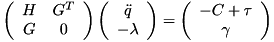, the velocity-level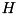, or the acceleration-level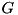. These constraints all end up being applied at the acceleration-level by taking successive derivatives until we are left with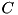. A new concrete Constraint class must provide implementations for the following methods:

• bind
• calcConstraintJacobian
• calcGamma
• calcPositionError
• calcVelocityError
• calcConstraintForces

Please see the doxygen for each of these functions in the Constraint interface for details. In addition, please have a look at Constraint_Contact (and ContactsTests.cc) and Constraint_Loop (and LoopConstraintsTests.cc) for working examples to guide your own implementation.

Please note that constraints are challenging to derive and implement even for multibody dynamics experts. If you are unfamiliar with the concept please refer to Featherstone's Robot Dynamics Algorithms text. If you are authoring a new constraint please read the section below on how to test your constraint.

• Physical correctness (and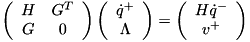): Workless? Ensure that the constraint is workless: perform a forward simulation of a passive system that includes the new constraint oscillating under gravity. Compute the sum of kinetic and potential energy called system energy. System energy should be constant, but will exhibit small amounts of accumulating error due to integration error. If the tolerances on the integration are tightened these errors should also drop. This kind of analysis is usually done when the first implementation of the constraint is being developed. This kind of test is usually not included as a unit test because it requires an accurate numerical integrator (which introduces an external dependency). A faster version of this test is to evaluate the forward dynamics of a given set of states and then sum up the joint powers which should sum to zero in a conservative system.
• Physical correctness (and): Agrees with equivalent joint-coordinate model? Evaluate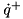by calling ForwardDynamicsConstraintsDirect on your system. If possible construct a model that uses joint coordinates that is perfectly equivalent to the model that uses constraints and evaluate its forward dynamics using the same initial state. The matching indices ofshould be identical. Examples of tests like this can be seen in ConstraintCorrectnessTest in LoopConstraintsTests.cc.
• Compare against static-equilibrum calculations (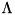) Set-up a simple test model for which you can easily compute the correct values for the position error, velocity error, and constraint forces. This is most easily done by solving for the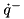that will put the sytem in a state of static equilibrium. Then you can easily solve by hand for the forces the constraint should develop and compare those to the values returned by calcForce. Examples of tests like this can be seen in TestExtendedConstraintFunctionsContact in ContactsTests.cc and TestExtendedConstraintFunctionsLoop in LoopConstraintsTests.cc
• Compare against known errors (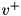and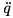) The values returned for the position and velocity errors of the constraint are most easily evaluated by constructing a system in which the constraint is satisfied at the position and velocity level and then calling calcPositionError and calcVelocityError to ensure that the returned errors are zero. A follow up test is then to introduce a known error to the generalized positions and velocities and sure that the error returned by cal Tests like this can be found in TestExtendedConstraintFunctionsContact in ContactsTests.cc and TestExtendedConstraintFunctionsLoop in LoopConstraintsTests.cc.

Definition at line 139 of file Constraint.h.

## ◆ ~Constraint()

 virtual ~Constraint ( )
inlinevirtual

Definition at line 366 of file Constraint.h.

## ◆ Constraint() [1/2]

 Constraint ( )

## ◆ Constraint() [2/2]

 Constraint ( const char * nameOfConstraint, unsigned int typeOfConstraint, unsigned int sizeOfConstraint, unsigned int userDefinedIdNumber )
inline
Parameters
 name (optional) name of the constraint. Use only as a means to find a specific constraint. typeOfConstraint corresponds to the enums listed in ConstraintTypes. This parameter is used by a few methods that only work with specific types of constraints such as ForwardDynamicsContactsKokkevis. sizeOfConstraint the number of equations that define the constraint manifold. Equivalently this is the number of rows in this constraints Jacobian. userDefinedIdNumber an integer that the user can set to rapidly retrieve this constraint from the set.

Definition at line 386 of file Constraint.h.

## Member Function Documentation

 void addInBaumgarteStabilizationForces ( const Math::VectorNd & errPosSys, const Math::VectorNd & errVelSys, Math::VectorNd & gammaSysOutput )
inline
Parameters
 errPosSys : the position error vector of the system errVelSys : the velocity error vector of the system gammaSysOutput the gamma vector of the system

Definition at line 507 of file Constraint.h.

 void addToConstraintSet ( const unsigned int rowIndex )
inline

This function is called by the functions in ConstraintSet that add a Constraint to the system. DO NOT TOUCH THIS.

Parameters
 rowIndex the first index of this constraint in the systems constraint Jacobian.

Definition at line 416 of file Constraint.h.

## ◆ bind()

 virtual void bind ( const Model & model )
pure virtual

Any local memory that has a dimension of N, where N is the length of QDot, is resized in this function.

Parameters
 model a reference to the multibody model. Use the model's fields of qdot_size to size local memory (if any) that depends on the model.

Implemented in ContactConstraint, and LoopConstraint.

## ◆ calcConstraintForces()

 virtual void calcConstraintForces ( Model & model, const double time, const Math::VectorNd & Q, const Math::VectorNd & QDot, const Math::MatrixNd & GSys, const Math::VectorNd & LagrangeMultipliersSys, std::vector< unsigned int > & constraintBodiesOutput, std::vector< Math::SpatialTransform > & constraintBodyFramesOutput, std::vector< Math::SpatialVector > & constraintForcesOutput, ConstraintCache & cache, bool resolveAllInRootFrame = false, bool updateKinematics = false )
pure virtual

This function resolves the generalized forces this constraint applies to the system into the wrenches that are applied to the bodies that are involved in the constraint.

Parameters
 model a reference to the multibody model. time the time which is included so that rheonomic constraints might be included (in the future). Q the vector of generalized positions. QDot the vector of generalized velocities. GSys a reference to the constraint Jacobian for the entire system. Ifis needed in this function do not re-evaluate it but instead extract it from the system: this constraint's sub-matrix begins at rowInSystem, has sizeOfConstraint rows, and the full number of columns of GSys. LagrangeMultipliersSys the vector of Lagrange multipliers for the entire system. The Lagrange multipliers for this constraint begin at rowInSystem and has sizeOfConstraint elements. constraintBodiesOutput contains the indices of the bodies that contain the local frames listed in constraintBodyFramesOutput. If resolveAllInRootFrame is true, all of these indicies should be set to 0: in this case all of the wrenches are resolved in the root frame. constraintBodyFramesOutput contains the local transformation from the origin of each body frame listed in constraintBodiesOutput to the frame in which the constraint wrench is applied. Note the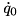frame is located on thebody. If resolveAllInRootFrame is true, all of these frames have their origins resolved in the global frame and their orientation set to match that tof the global frame. constraintForcesOutput contains the wrenches that the constraint applies to the local frames listed in constraintBodyFramesOutput. Note theforce is resolved in theframe.If resolveAllInRootFrame is true, this wrench should be rotated into the coordinates of the root frame. cache a ConstraintCache object which contains ample pre-allocated memory that can be used to reduce the memory footprint of each Constraint implementation. resolveAllInRootFrame When this parameter is - false: the wrenches are resolved into their local frames. - true: the wrenches are resolved into coordinates of the global frame  updateKinematics setting this flag to true will cause all calls to kinematic dependent functions to be updated using the generalized coordinates passed into this function.

Implemented in ContactConstraint, and LoopConstraint.

## ◆ calcConstraintJacobian()

 virtual void calcConstraintJacobian ( Model & model, const double time, const Math::VectorNd & Q, const Math::VectorNd & QDot, Math::MatrixNd & GSysOutput, ConstraintCache & cache, bool updateKinematics = false )
pure virtual

In this function the matrixof this contraint is inserted into the system constraint Jacobian(GSysOutput). The submatrixbegins at rowInSystem, has sizeOfConstraint number of rows, and fills the full number of columns in G.

Parameters
 model a reference to the multibody model. time the time which is included so that rheonomic constraints might be included (in the future). Q the vector of generalized positions. QDot the vector of generalized velocities. GSysOutput a reference to the constraint Jacobian for the entire system. Insert the G sub-matrix for this constraint beginning and rowInSystem, with a length sizeOfConstraint rows, and a width of the full number of columns in GSysOutput. cache a ConstraintCache object which contains ample pre-allocated memory that can be used to reduce the memory footprint of each Constraint implementation. updateKinematics setting this flag to true will cause all calls to kinematic dependent functions to be updated using the generalized coordinates passed into this function.

Implemented in ContactConstraint, and LoopConstraint.

## ◆ calcGamma()

 virtual void calcGamma ( Model & model, const double time, const Math::VectorNd & Q, const Math::VectorNd & QDot, const Math::MatrixNd & GSys, Math::VectorNd & gammaSysOutput, ConstraintCache & cache, bool updateKinematics = false )
pure virtual

In this function the vectorof this constraint is inserted into the right-hand-side vectorof the system. The vectorbegins at rowInSystem, and has sizeOfConstraint rows.

Parameters
 model a reference to the multibody model. time the time which is included so that rheonomic constraints might be included (in the future). Q the vector of generalized positions. QDot the vector of generalized velocities. GSys a reference to the constraint Jacobian for the entire system. Ifis needed in this function do not re-evaluate it but instead extract it from the system. gammaSysOutput the system's gamma vector. Insert the gamma sub-vector for this constraint beginning and rowInSystem and with a length of sizeOfConstraint rows. cache a ConstraintCache object which contains ample pre-allocated memory that can be used to reduce the memory footprint of each Constraint implementation. updateKinematics setting this flag to true will cause all calls to kinematic dependent functions to be updated using the generalized coordinates passed into this function.

Implemented in ContactConstraint, and LoopConstraint.

## ◆ calcPositionError()

 virtual void calcPositionError ( Model & model, const double time, const Math::VectorNd & Q, Math::VectorNd & errSysOutput, ConstraintCache & cache, bool updateKinematics = false )
pure virtual

In this function the sub vectorof this constraint is inserted into the position error vector of the system. If the constraint is velocity-level constraint or higher (noted in the boolean member variable positionConstraint) this should be set to zero. The vectorbegins at rowInSystem, and has sizeOfConstraint rows.

Parameters
 model a reference to the multibody model time the time which is included so that rheonomic constraints might be included (in the future). Q the vector of generalized positions. errSysOutput the system's constraint position error vector. Insert the position error sub-vector for this constraint beginning and rowInSystem and with a length of sizeOfConstraint rows. cache a ConstraintCache object which contains ample pre-allocated memory that can be used to reduce the memory footprint of each Constraint implementation. updateKinematics setting this flag to true will cause all calls to kinematic dependent functions to be updated using the generalized coordinates passed into this function.

Implemented in ContactConstraint, and LoopConstraint.

## ◆ calcVelocityError()

 virtual void calcVelocityError ( Model & model, const double time, const Math::VectorNd & Q, const Math::VectorNd & QDot, const Math::MatrixNd & GSys, Math::VectorNd & derrSysOutput, ConstraintCache & cache, bool updateKinematics = false )
pure virtual

In this function the sub vectorof this constraint is inserted into the position error vector of the system. If the constraint is an acceleration-level constraint (noted in the boolean member variable velocityConstraint) this sub vector should be zero. The vectorbegins at rowInSystem, and has sizeOfConstraint rows.

Parameters
 model a reference to the multibody model. time the time which is included so that rheonomic constraints might be included (in the future). Q the vector of generalized positions. QDot the vector of generalized velocities. GSys a reference to the constraint Jacobian for the entire system. Ifis needed in this function do not re-evaluate it but instead extract it from the system: this constraint's sub-matrix begins at rowInSystem, has sizeOfConstraint rows, and the full number of columns of GSys. derrSysOutput the system's constraint velocity error vector. Insert the velocity error sub-vector for this constraint beginning and rowInSystem and with a length of sizeOfConstraint rows. cache a ConstraintCache object which contains ample pre-allocated memory that can be used to reduce the memory footprint of each Constraint implementation. updateKinematics setting this flag to true will cause all calls to kinematic dependent functions to be updated using the generalized coordinates passed into this function.

Implemented in ContactConstraint, and LoopConstraint.

## ◆ enableConstraintErrorFromAccelerationLevel() [1/2]

 void enableConstraintErrorFromAccelerationLevel ( )
inline

: Will set the all elements in positionConstraint and velocityConstraint to be consistent with a constraint that is described from the acceleration-level up.

Definition at line 653 of file Constraint.h.

## ◆ enableConstraintErrorFromAccelerationLevel() [2/2]

 void enableConstraintErrorFromAccelerationLevel ( unsigned int constraintSubIndex )
inline

: Will set the vectors positionConstraint and velocityConstraint to be consistent with a constraint that is described from the acceleration-level: both position and velocity errors will be set to zero.

Parameters
 constraintSubIndex the sub index into this contraint group.

Definition at line 615 of file Constraint.h.

## ◆ enableConstraintErrorFromPositionLevel() [1/2]

 void enableConstraintErrorFromPositionLevel ( )
inline

: Will set the all elements in positionConstraint and velocityConstraint to be consistent with a constraint that is described from the position-level up.

Definition at line 627 of file Constraint.h.

## ◆ enableConstraintErrorFromPositionLevel() [2/2]

 void enableConstraintErrorFromPositionLevel ( unsigned int constraintSubIndex )
inline

: Will set the vectors positionConstraint and velocityConstraint to be consistent with a constraint that is described from the position-level up.

Parameters
 constraintSubIndex the sub index into this contraint group.

Definition at line 584 of file Constraint.h.

## ◆ enableConstraintErrorFromVelocityLevel() [1/2]

 void enableConstraintErrorFromVelocityLevel ( )
inline

: Will set the all elements in positionConstraint and velocityConstraint to be consistent with a constraint that is described from the velocity-level up.

Definition at line 640 of file Constraint.h.

## ◆ enableConstraintErrorFromVelocityLevel() [2/2]

 void enableConstraintErrorFromVelocityLevel ( unsigned int constraintSubIndex )
inline

: Will set the vectors positionConstraint and velocityConstraint to be consistent with a constraint that is described from the velocity-level up: position errors will be set to zero while velocity errors will be computed.

Parameters
 constraintSubIndex the sub index into this contraint group.

Definition at line 599 of file Constraint.h.

## ◆ getBaumgarteStabilizationForces()

 void getBaumgarteStabilizationForces ( const Math::VectorNd & errPos, const Math::VectorNd & errVel, Math::VectorNd & baumgarteForces )
inline
Parameters
 errPos : the position error vector of this constraint errVel : the velocity error vector of this constraint baumgarteForces the Baumgarte stabilization forces

Definition at line 493 of file Constraint.h.

## ◆ getBaumgarteStabilizationParameters()

 void getBaumgarteStabilizationParameters ( Math::Vector2d & bgParamsUpd )
inline
Parameters
 bgParamsUpd (output) the Baumgarte stabilization coefficients for this constraint. Note the velocity error coefficient is in the 0 index and the position error coefficient is in the 1st index.

Definition at line 484 of file Constraint.h.

## ◆ getBodyFrames()

 const std::vector< Math::SpatialTransform > & getBodyFrames ( )
inline
Returns
a vector of local frames (which are located on the corresponding body in the vector of body Ids returned by getBodyIds) that are involved in this constraint.

Definition at line 703 of file Constraint.h.

## ◆ getBodyIds()

 const std::vector< unsigned int > & getBodyIds ( )
inline
Returns
a vector of the bodyIds that are involved in this constraint

Definition at line 694 of file Constraint.h.

## ◆ getConstraintIndex()

 unsigned int getConstraintIndex ( )
inline
Returns
the index of the first row in G in which this constraint's entries appear.

Definition at line 541 of file Constraint.h.

## ◆ getConstraintJacobian()

 void getConstraintJacobian ( const Math::MatrixNd & GSys, Math::MatrixNd & GConstraint )
inline
Parameters
 GSys : a reference to the system's constraint Jacobian GConstraint : a reference to this constraint's entry within the system constraint Jacobian

Definition at line 441 of file Constraint.h.

References MX_Xd_dynamic::block(), and MX_Xd_dynamic::cols().

## ◆ getConstraintSize()

 unsigned int getConstraintSize ( )
inline
Returns
the number of constraint equations in this constraint

Definition at line 533 of file Constraint.h.

## ◆ getConstraintType()

 unsigned int getConstraintType ( )
inline
Returns
the type of this constraint as defined in the struct ConstraintType

Definition at line 526 of file Constraint.h.

## ◆ getGamma()

 void getGamma ( const Math::VectorNd & gammaSys, Math::VectorNd & gammaConstraint )
inline
Parameters
 gammaSys a reference to the system's gamma vector gammaConstraint a reference to this constraint's entry within the system gamma vector

Definition at line 452 of file Constraint.h.

References MX_Xd_dynamic::block().

## ◆ getName()

 const char * getName ( )
inline
Returns
the user defined name

Definition at line 687 of file Constraint.h.

## ◆ getPositionError()

 void getPositionError ( const Math::VectorNd & errSys, Math::VectorNd & errConstraint )
inline
Parameters
 errSys a reference to the system's constraint position error errConstraint a reference to this constraint's entry within the system constraint position error.

Definition at line 462 of file Constraint.h.

References MX_Xd_dynamic::block().

## ◆ getPositionLevelError()

 bool getPositionLevelError ( unsigned int constraintSubIndex )
inline

Returns the boolean value that determines whether or not position-level errors are computed for this sub-index into this constraint.

Parameters
 constraintSubIndex the sub index of interest in this constraint

Definition at line 668 of file Constraint.h.

## ◆ getUserDefinedId()

 unsigned int getUserDefinedId ( )
inline
Returns
(optional) user-defined-id

Definition at line 425 of file Constraint.h.

## ◆ getVelocityError()

 void getVelocityError ( const Math::VectorNd & derrSys, Math::VectorNd & derrConstraint )
inline
Parameters
 derrSys a reference to the system's constraint velocity error derrConstraint a reference to this constraint's entry within the system constraint velocity error.

Definition at line 473 of file Constraint.h.

References MX_Xd_dynamic::block().

## ◆ getVelocityLevelError()

 bool getVelocityLevelError ( unsigned int constraintSubIndex )
inline

Returns the boolean value that determines whether or not velocity-level errors are computed for this sub-index into this constraint.

Parameters
 constraintSubIndex the sub index of interest in this constraint

Definition at line 679 of file Constraint.h.

## ◆ isBaumgarteStabilizationEnabled()

 bool isBaumgarteStabilizationEnabled ( )
inline
Returns
true if Baumgarte stabilization is enabled, false otherwise.

Definition at line 573 of file Constraint.h.

## ◆ setBaumgarteTimeConstant()

 void setBaumgarteTimeConstant ( double tStab )
inline

Calculates and sets the Baumgarte stabilization coefficients as a function of tStab, the approximate time-constant of stabilization.

Parameters
 tStab : a time-constant that is used to compute values for the position and velocity level stabilization terms. Note that a smaller time constant means stronger stabilization forces and a numerically stiffer system.

Definition at line 555 of file Constraint.h.

## ◆ setEnableBaumgarteStabilization()

 void setEnableBaumgarteStabilization ( bool flagEnableBaumgarteStabilization )
inline
Parameters
 flagEnableBaumgarteStabilization setting this to true will enable Baumgarte stabilization for this constraint.

Definition at line 566 of file Constraint.h.

## ◆ setUserDefinedId()

 void setUserDefinedId ( unsigned int userDefinedId )
inline
Parameters
 userDefinedId (optional) integer id for this constraint

Definition at line 432 of file Constraint.h.

## ◆ baumgarteEnabled

 bool baumgarteEnabled
protected

A flag which enables or disables Baumgarte stabilization.

Definition at line 733 of file Constraint.h.

## ◆ baumgarteParameters

 Math::Vector2d baumgarteParameters
protected

The Baumgarte stabilization coefficients at the position and velocity level

Definition at line 730 of file Constraint.h.

## ◆ bodyFrames

 std::vector< Math::SpatialTransform > bodyFrames
protected

Transform from the frame of the predecessor body to the constraint frame.

Definition at line 726 of file Constraint.h.

## ◆ bodyIds

 std::vector< unsigned int > bodyIds
protected

The index of the predecessor body in the vector of bodies in Model.

Definition at line 723 of file Constraint.h.

## ◆ id

 unsigned int id
protected

A user defined id which is unique to this constraint set.

Definition at line 712 of file Constraint.h.

## ◆ name

 std::string name
protected

A user defined name which is unique to this constraint set.

Definition at line 709 of file Constraint.h.

## ◆ positionConstraint

 std::vector< bool > positionConstraint
protected

A mask that is used to selectively enable/disable the calculation of position-level and velocity-level errors. These errors are used to functions that assemble the system at the position and velocity levels, and also by functions that stablize the constraint error at the position and velocity level.

Definition at line 740 of file Constraint.h.

## ◆ rowInSystem

 unsigned int rowInSystem
protected

The first row in G that corresponds to this constraint.

Definition at line 720 of file Constraint.h.

## ◆ sizeOfConstraint

 unsigned int sizeOfConstraint
protected

The number of rows that this constraint adds to G.

Definition at line 717 of file Constraint.h.

## ◆ typeOfConstraint

 unsigned int typeOfConstraint
protected

The type of this constraint.

Definition at line 714 of file Constraint.h.

## ◆ velocityConstraint

 std::vector< bool > velocityConstraint
protected

Definition at line 741 of file Constraint.h.

The documentation for this class was generated from the following file: